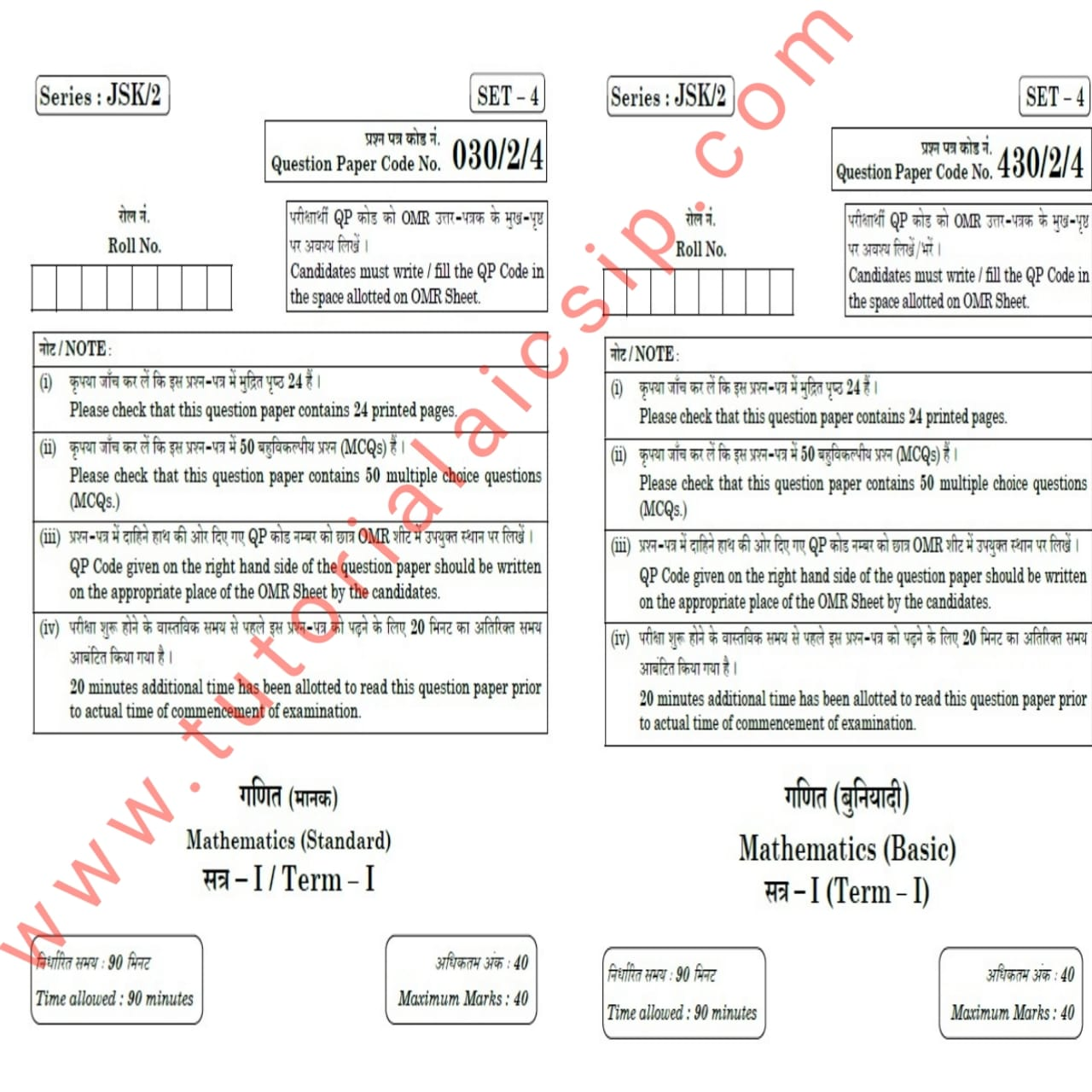In this article, we are going to provide you with the CBSE Term 1 Maths Answer Key 2021 Class 10 (Standard and Basic). So let’s start. CBSE conducted Mathematics Standard (041) and Maths Basic term 1 board exam on 04.12.2021. Students of Maths standard and maths basic of class 10 are searching for the CBSE Term 1 Maths Answer Key 2021 Class 10 (Standard and Basic). Here you will get it.

Topics Covered

## CBSE class 10 Maths Term 1 Answer Key

The basic details about the CBSE class 10 maths questions paper are as follows:

## CBSE Term 1 Maths Answer Key 2021 Class 10 (Standard and Basic)

Check your answers from the following. This answer key is prepared after a question papers discussion with subject experts and teachers who are teaching class 10 maths.

The following is the answers for Maths Basic. The question paper code is 430/2/4, set 4, the series is JSK/2.